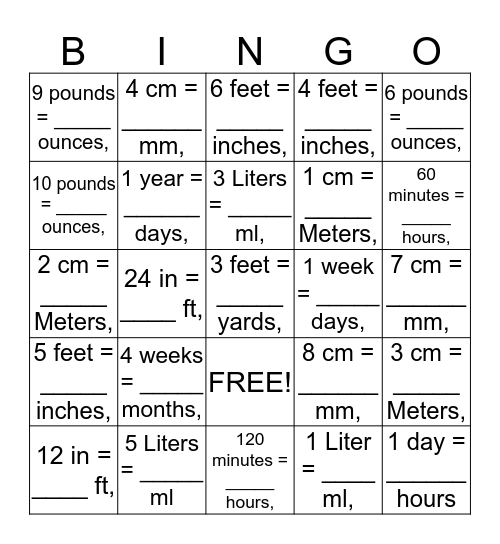# Math ConversionThis bingo card has 26 words: FREE!, 1 Liter = ____ ml,, 3 Liters = _____ ml,, 5 Liters = _____ ml, 6 pounds = _____ ounces,, 9 pounds = _____ ounces,, 10 pounds = _____ ounces,, 4 cm = ______ mm,, 7 cm = ______ mm,, 8 cm = ______ mm,, 1 cm = _____ Meters,, 2 cm = _____ Meters,, 3 cm = _____ Meters,, 12 in = ____ ft,, 24 in = ____ ft,, 36 in = ____ ft,, 4 feet = _____ inches,, 5 feet = _____ inches,, 6 feet = _____ inches,, 3 feet = _____ yards,, 60 minutes = _____ hours,, 120 minutes = _____ hours,, 1 week = _____ days,, 4 weeks = _____ months,, 1 year = ______ days, and 1 day = ______ hours.

## Play Online